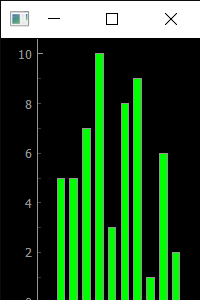GeeksforGeeks App
Open AppBrowser
Continue

# PyQtGraph – Setting Minimum Size of Plot Window

In this article we will see how we can set minimum size of the plot window in the PyQtGraph module. PyQtGraph is a graphics and user interface library for Python that provides functionality commonly required in designing and science applications. Its primary goals are to provide fast, interactive graphics for displaying data (plots, video, etc.) and second is to provide tools to aid in rapid application development (for example, property trees such as used in Qt Designer).Plot windows consist of two main parts: the Plot Panel containing the actual plotted graphics and the Control Panel. By default plot window can be resized, by setting minimum size we assure that user will not be able to resize the window below the minimum size point. We can create a plot window with the help of command given below

```# creating a pyqtgraph plot window
window = pg.plot()```

In order to do this we use setMinimumSize method with the plot window object Syntax : window.setMinimumSize(width, height) Argument : It takes two integer as argument Return : It returns None

Below is the implementation

## Python3

 `# importing pyqtgraph as pg``import` `pyqtgraph as pg` `# importing QtCore and QtGui from the pyqtgraph module``from` `pyqtgraph.Qt ``import` `QtCore, QtGui` `# importing numpy as np``import` `numpy as np` `import` `time` `# creating a pyqtgraph plot window``window ``=` `pg.plot()` `# setting window geometry``# left = 100, top = 100``# width = 600, height = 500``window.setGeometry(``100``, ``100``, ``600``, ``500``)` `# title for the plot window``title ``=` `"GeeksforGeeks PyQtGraph"` `# setting window title to plot window``window.setWindowTitle(title)` `# create list for y-axis``y1 ``=` `[``5``, ``5``, ``7``, ``10``, ``3``, ``8``, ``9``, ``1``, ``6``, ``2``]` `# create horizontal list i.e x-axis``x ``=` `[``1``, ``2``, ``3``, ``4``, ``5``, ``6``, ``7``, ``8``, ``9``, ``10``]` `# create pyqt5graph bar graph item``# with width = 0.6``# with bar colors = green``bargraph1 ``=` `pg.BarGraphItem(x ``=` `x, height ``=` `y1, width ``=` `0.6``, brush ``=``'g'``)` `# add item to plot window``# adding bargraph item to the window``window.addItem(bargraph1)` `# setting minimum size of plot window``window.setMinimumSize(``200``, ``300``)`   `# main method``if` `__name__ ``=``=` `'__main__'``:``    ` `    ``# importing system``    ``import` `sys``    ` `    ``# Start Qt event loop unless running in interactive mode or using``    ``if` `(sys.flags.interactive !``=` `1``) ``or` `not` `hasattr``(QtCore, ``'PYQT_VERSION'``):``        ``QtGui.QApplication.instance().exec_()``       `

Output : Below is the minimum size which can window can attainMy Personal Notes arrow_drop_up